# Complex numbers in pure Snap!

As the title says.

Project here.

Currently incomplete.

How do I do complex number division?

$${(a+bi)\over(c+di)} = {{{(a+bi)\cdot(c-di)}\over {(c+di)\cdot(c-di)}}} = {{{(a+bi)\cdot(c-di)}}\over c^2+d^2}$$
and now the denominator is a real number.

Vocabulary: $$c-di$$ is called the complex conjugate of $$c+di$$. You can see why it's a concept that deserves a name from the above example.

Actually, the denominator is c^2+d^2.

Oops! Fixed.

Yes, I knew that.

But I need it so that neither the numerator nor the denominator are complex

???
The above expression rewritten as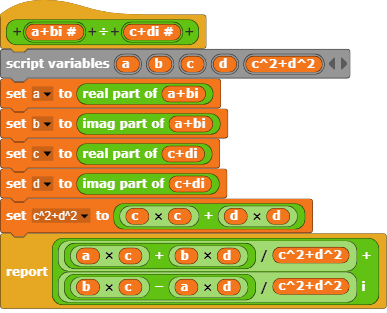gives a correct results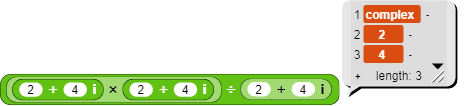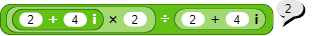So I don't have to divide a complex number by a real number and either get a nonsensical answer (using the primitive) or an infinite loop (using the re-written version). But I figured out that I can take the reciprocal of the real denominator and multiply it with the complex number, using $${a\over b}=a\times{1\over b}$$.

Is this right?: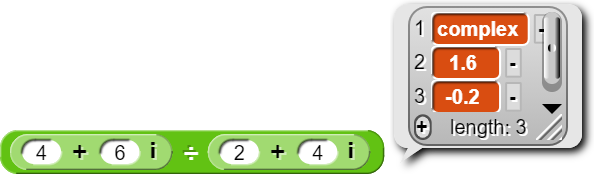If you load the Bignums library, you get complex arithmetic, so you can check your results against theirs.

Umm, if the result of the division isn't real, then the numerators won't be real either. But division distributes over addition, so
$$(a+bi)\div denom = (a/denom) +(b/denom)i$$
and those two divisions are of real numbers.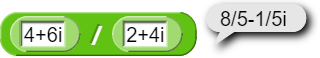I didn't do that, but rather

which seems to work.

Sure, dividing is the same as multiplying by the reciprocal.

...And, in this case, you got it so the denominator doesn't have an imaginary part, so I was multiplying a complex number by the reciprocal of a real number, which I could do, because I had multiplication working.

Now how do I take a complex number to the power of another complex number?

$$c^{a+bi}=c^a\cdot c^{bi} = c^a \cdot (\cos b+i\sin b)$$
where $$c$$ is a complex number.

I need to turn $$a+bi^{c+di}$$ into something where the only things being raised to a power are reals raised to reals.

Right, but I've reduced the problem for you from complexcomplex to complexreal. For the latter, I think your best bet is to convert the base to polar form:
$$a+bi = r \cdot (\cos \theta + i \sin \theta)$$ where
$$r=\sqrt{a^2+b^2}$$, $$\theta=\arctan (b/a)$$ (in radians).
Then you have realreal for $$r$$, and umm I think
$$(\cos \theta +i\sin\theta)^x=\cos x\theta +i\sin x\theta$$
but you should check in Wikipedia.

I don't like trigonometric functions: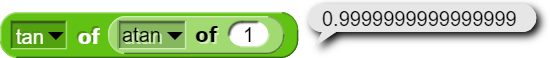floatingpoint problemsuse big nums(only the use bignums block)